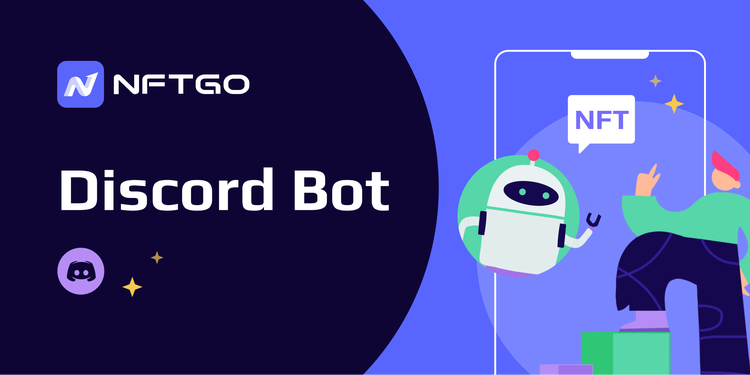The Ultimate Guide to NFTGo’s New Rarity Model | NFTGo Research

# The Ultimate Guide to NFTGo’s New Rarity ModelNFTGo Research
NFTGo Research
Jan 12, 2022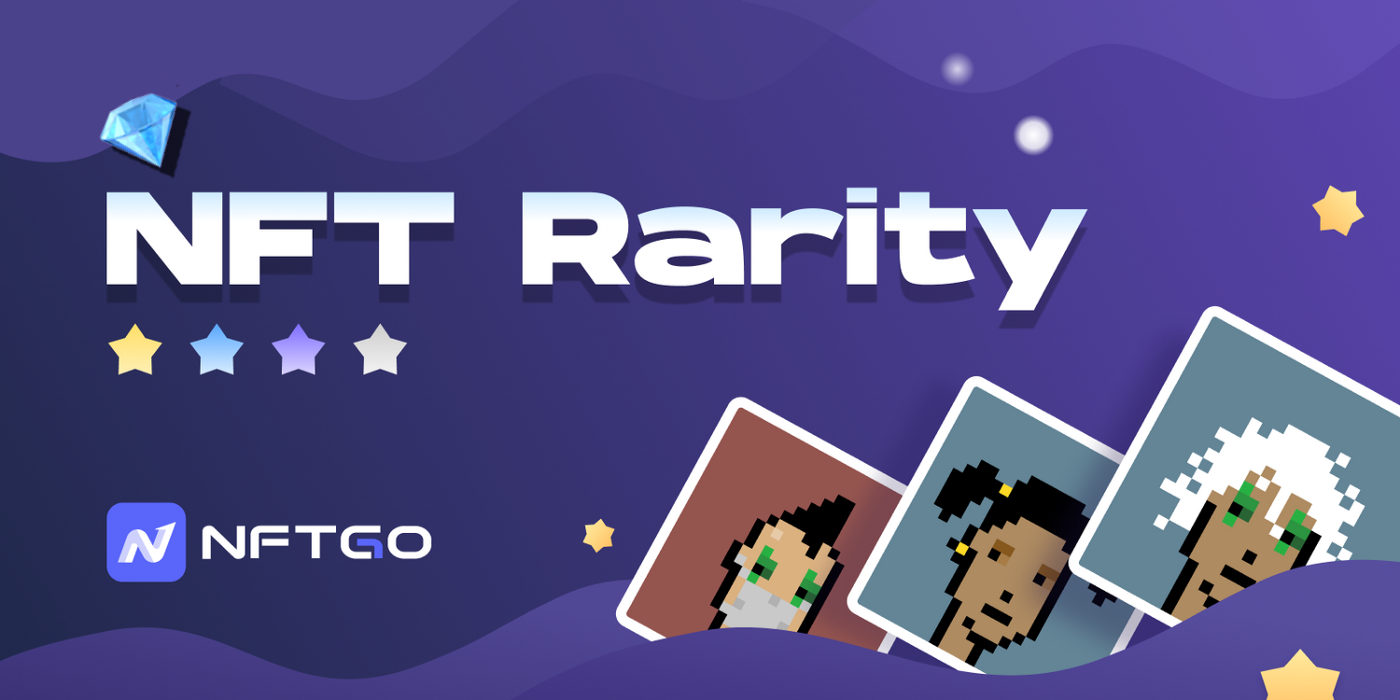You might have just paid 20K for an NFT, but was it worth it?

NFTs come with a variety of attributes and they get high valuations for their uniqueness. But how do we find out what’s unique?

In general, synonyms of rarity are special and unique. Essentially, the rarity of an object depends largely on its differences from other objects: the greater the difference is, the more special and rarer this object will be. Quantifying the combined difference between an object and other things within the group reflects the object’s rarity. Based on this logic, NFTGO uses a statistical approach called Jaccard distance to evaluate the real rarity of NFTs.

What is Jaccard distance?

NFTGO’s innovative rarity evaluation model goes beyond the mainstream approaches employed by products like rarity.tools. The common way of approaching NFT rarity is flawed and it has a low accuracy in some cases. An example of an inaccurate prediction from these models is the rarity score for Ape #947 from the Bored apes club. Rarity.tools gave a rarity score of 168.23. Even though the NFT does not seem to have any unique attributes.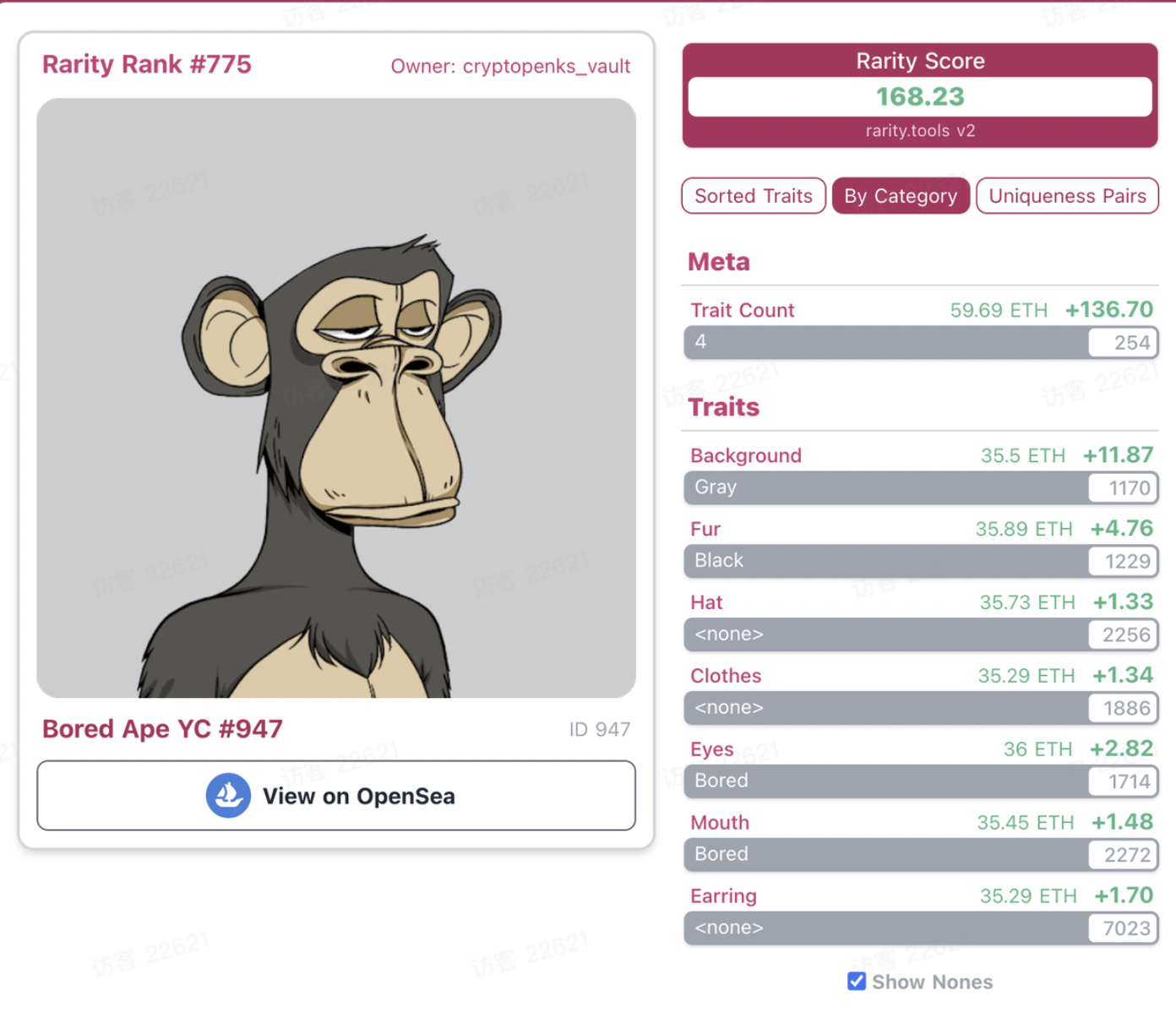The reason is that these models naively add up the traits and the frequency of occurrence for each of the traits. This simple approach doesn’t take into account how each trait contributes to an NFT’s uniqueness. This is why NFTGO has created a complex model that takes a more scientific approach to estimating an NFT’s rarity.

Jaccard distance is a metric for measuring similarities between two sets of data. In our case, the Jaccard distance takes into account the similarities between NFTs based on their attributes and assigns a rarity score ranging from 0 to 100.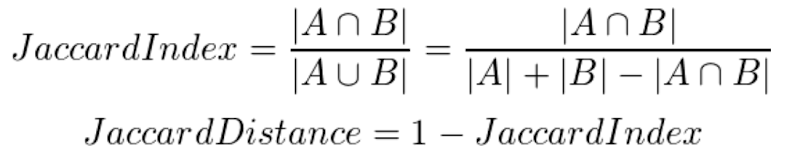The distance between two data points, in this case, is equal to the number of similar attributes divided by the total number of their attributes. This number is subtracted by one to get the Jaccard distance.

We see how this plays out in different NFTs. Our model employs this algorithm to quantitatively measure the similarities between two NFTs. This opens up the possibility for us to estimate an NFT’s rarity scientifically.

Deeper dive

This approach measures the overlap between two finite sets. The higher the overlap, the lower the Jaccard distance. By understanding the similarities across all the NFTs in a collection, we can gauge how rare an NFT is. For evaluating an NFT’s rarity, we compare it to all the other NFTs in the collection. For each NFT pair, we take the Jaccard distance and normalize the results to get the optimal score.

The process of calculating an NFT’s rarity contains four steps:

Step 1) Dividing the number of similar traits by the total number of unique attributes. Then, subtracting the results from one. (We repeat this process for all the NFT pairs)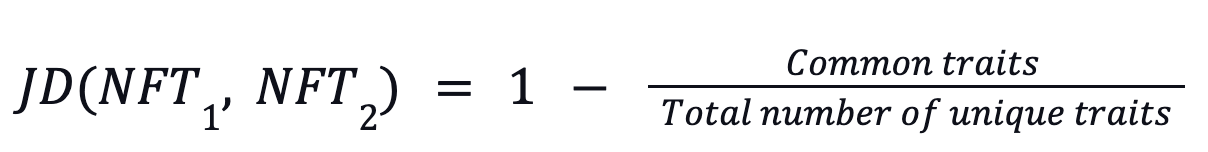Step 2) Taking the average of all the resultsStep 3) Normalizing the results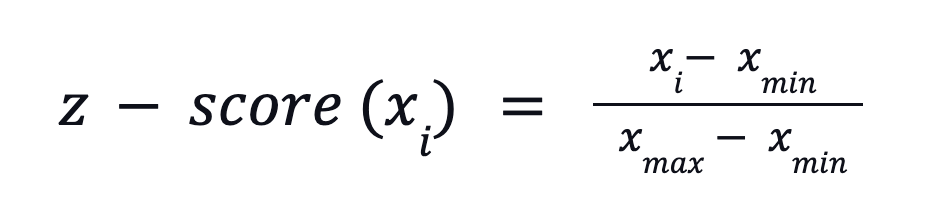Step 4) Multiplying the z-score by 100

CryptoPunks: case study

For understanding the model on a deeper level, let’s consider three NFTs from the CryptoPunks collection:

Punk#6089 { Alien, Knitted Cap, Earing},

Punk#7523 {Alien, Medical Mask, Knitted Cap, Earring}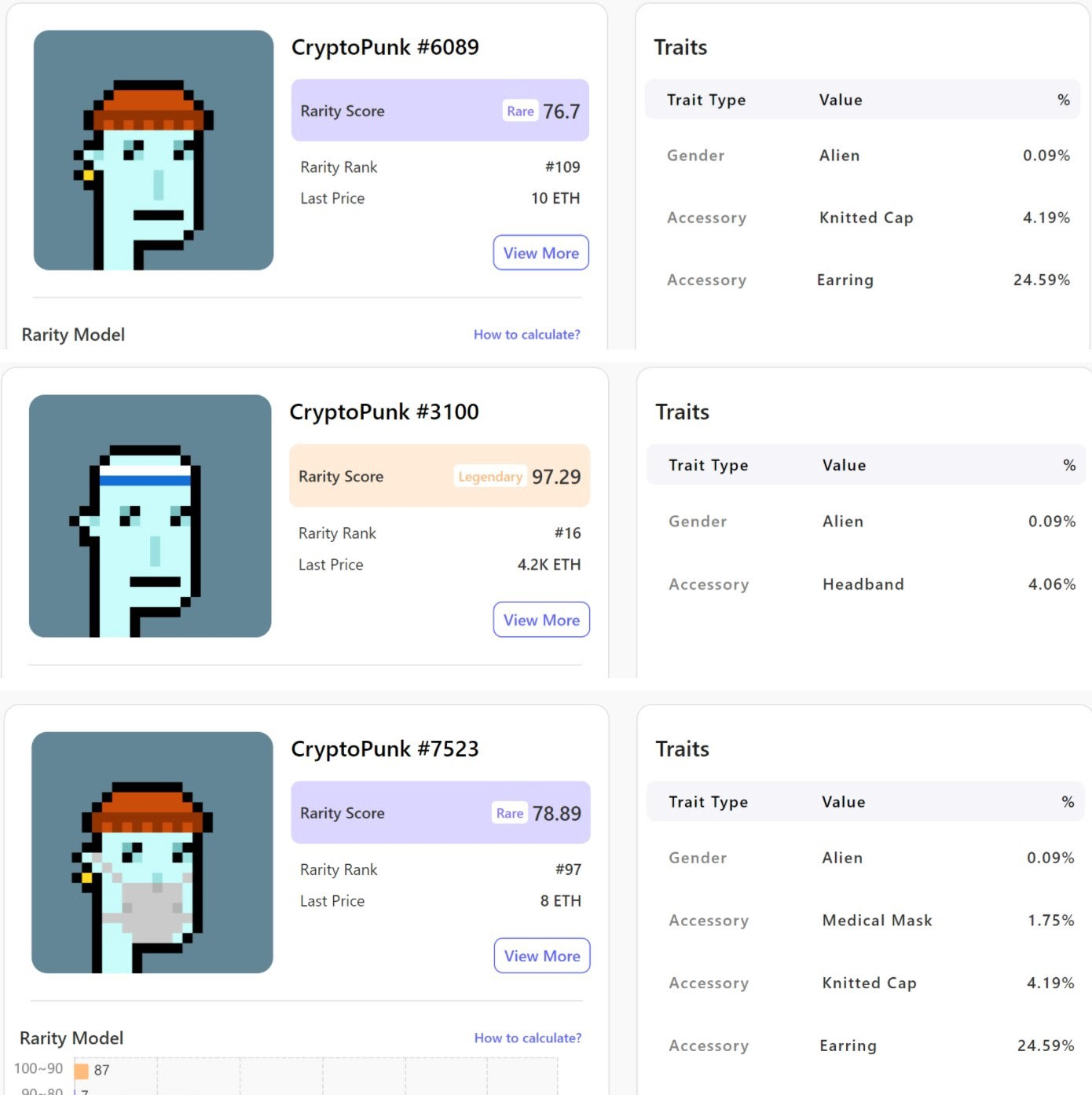We want to know how “rare” Punk#6089 is relative to Punk #3100 and Punk #7523.

We can do this by first calculating the Jaccard distance for #6089 and #3100, and then doing the same for #6089 and #7523. Take the average and normalize the results.

We can see that #6089 and #3100 have a total of 4 unique traits. The NFTs have 1 trait in common.

JD for #6089 and #3100:

J(#6089, #3100) =1–0.25= 0.75

Now we perform the same calculation for #6089 and #7523:

They have three values in common.

There’s a total of 4 unique values

J(#6089, #7523) = 1–0.75 = 0.25

The average of the JD for #6089 and other two punks:

Average = (0.75 + 0.25 ) / 2 = 0.5

If we calculate the average JD for #3100 and #7523, we get 0.775 and 0.525 respectively.

We normalize the final average using this formula, later on, we will see how crucial this formula is. By applying this normalization, we get the z-score for the NFT.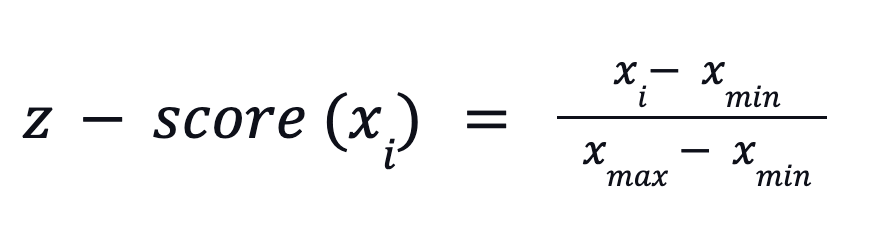For the normalization step to get #6089’s z-score, we get the difference between the initial value we got for #6089 from step 2 and the lowest average value of all the dataset. In this case, this would be its own average JD. Then we divide the results by the difference between the maximum and minimum values in the dataset.

z(A) = (0.5–0.5 ) / (0.775–0.5) = 0

Finally, we multiply the z-score by 100 to get the rarity score. This is:

#6089: 0

#3100: 100

#7523: 9

We can conclude that #3100 is the rarest Punk and #6089 is the least rare in this collection of 3 sets. This ranking is also present in the actual model. This is just an abstraction of what the model is doing.

In our example, the sample size is only 3 but the real model’s sample size is 10,000. This is why our numbers are different from the model’s rankings.

Why does Jaccard distance work?

You now understand how the model transforms the data and gives you the final results. Now that it’s not a black box anymore, we can go back to the original question of “Why does it work”. This is more clear when we add some examples. This time, from the real NFT world!

Let’s go back to CryptoPunks. This collection has 10,000 NFTs in total. Our goal is to estimate the Rarity of a single NFT in this collection relative to the other NFTs. Keep in mind that rarity is a relative attribute and in this model, we consider all the data from the collection to indicate an NFT’s rarity.

Let’s look at CryptoPunk #5577. This Punk has 2 attributes in total. Some of them might commit to its rarity, others might bring its score lower. Let’s see how rare this NFT is.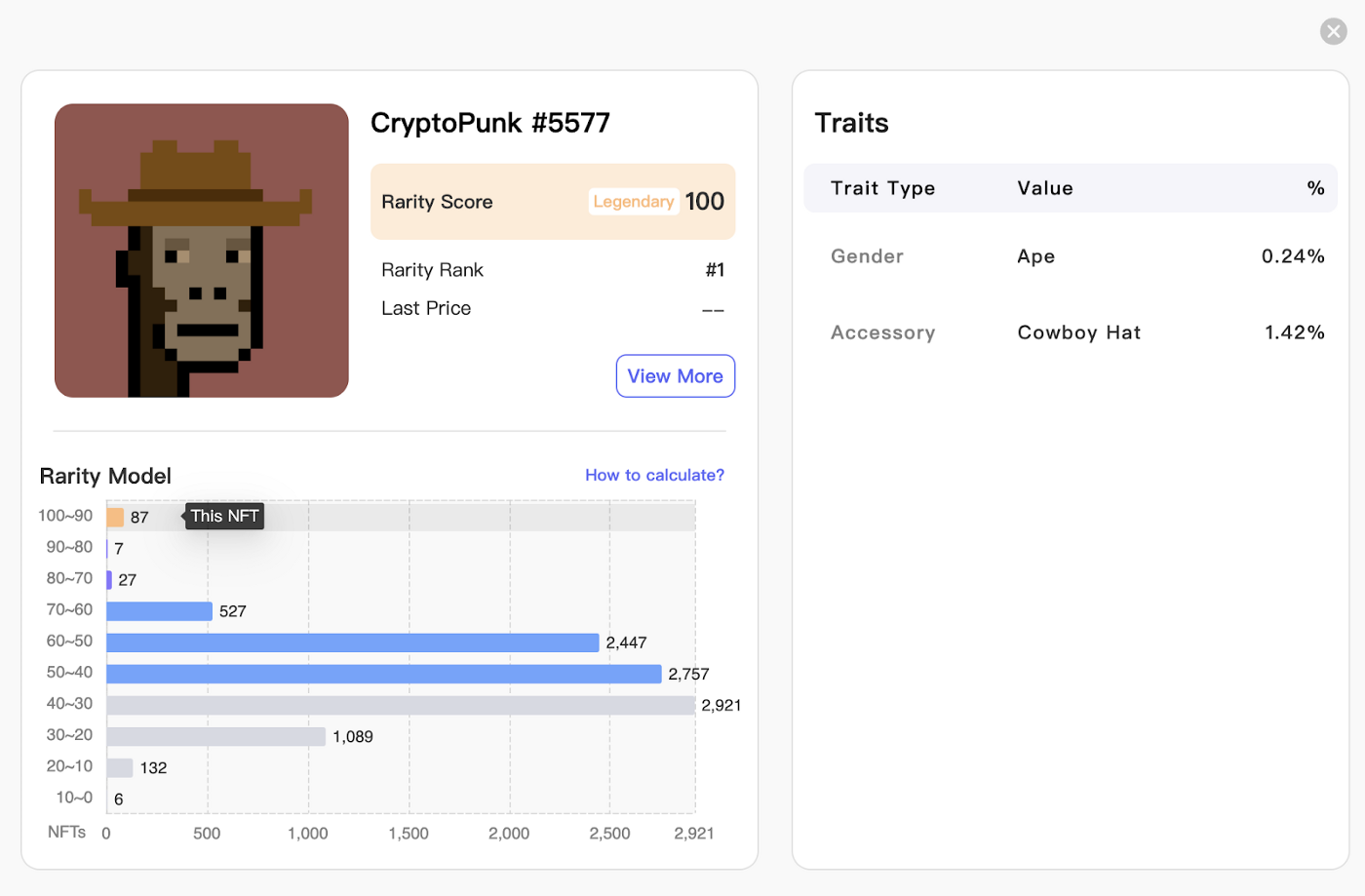A good way of visualizing the model’s point of view is using Venn diagrams. We compare the other 6968 NFTs with this Punk and get its Jaccard distance for each of them, as an example, here’s the computation for #5577 and #6965.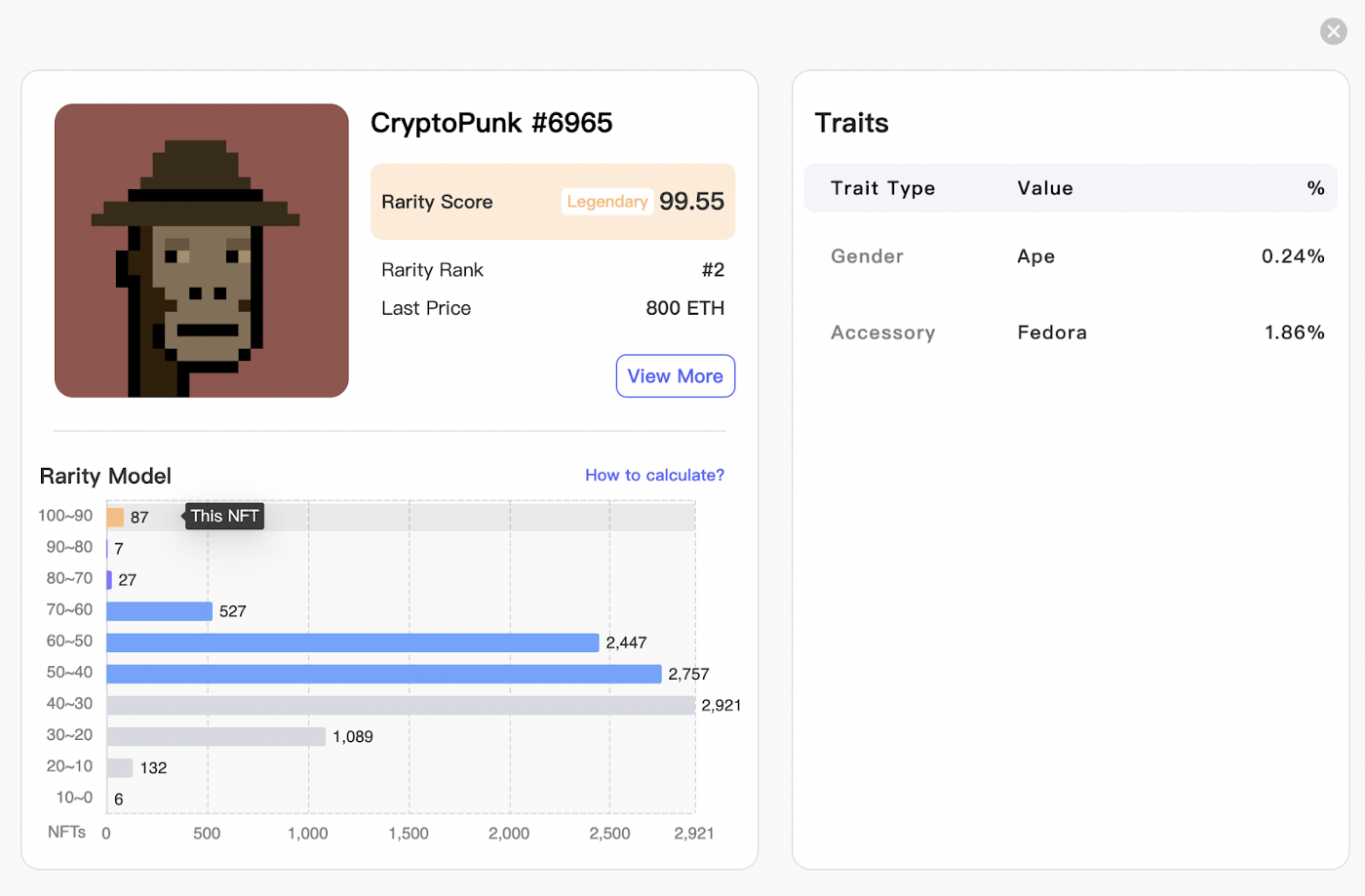You can see the rarity score for each of them -which was computed by the model-, and also a Venn diagram of their attributes.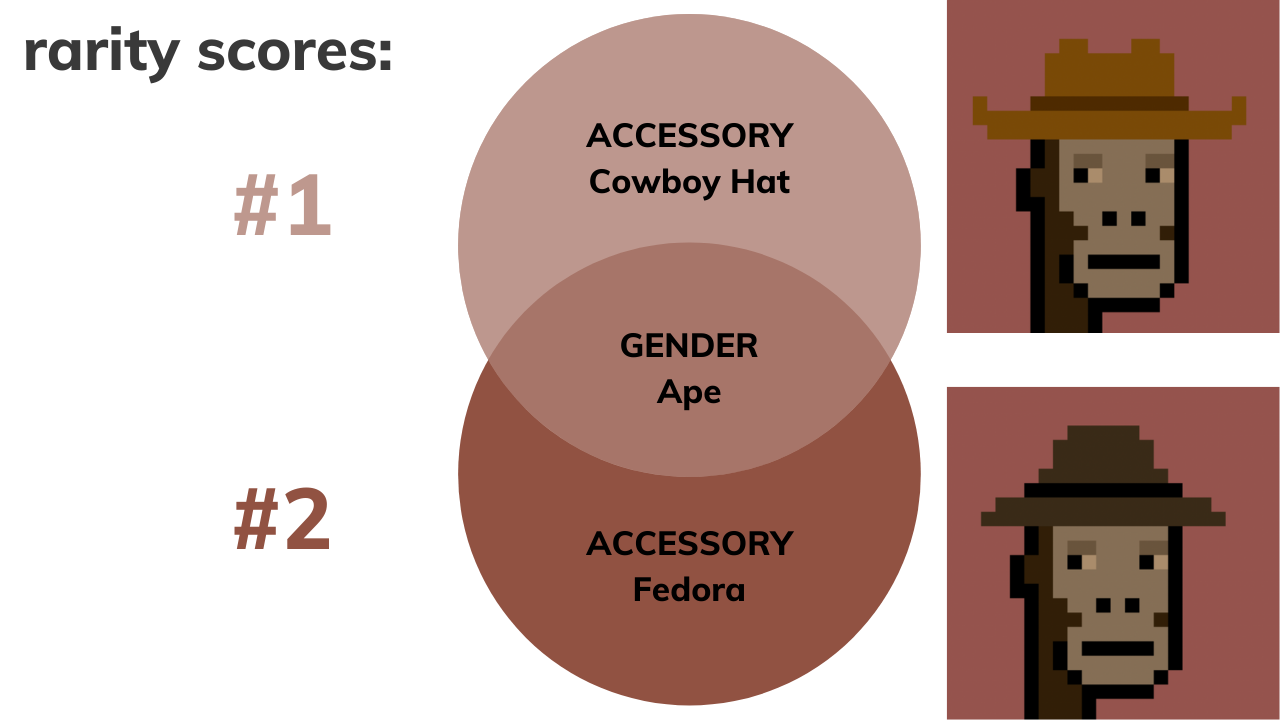The Jaccard distance for these two would be 1–1 / 3 which is about 0.7. Remember that we divide the number of unique attributes by the total number of attributes.

We can see that these two NFTs are relatively similar but not quite. The more similar the two NFTs are, the closer the Jaccard distance is to zero. The extreme case would be that if two NFTs have Identical attributes, they would have a Jaccard distance of 0 and therefore, none of them are “rare” relative to each other.

This calculation is spread across all NFT pairs in the collection. By taking the average, we can see how rare the NFT is relative to all the other NFTs in the collection.

But taking the average is not enough, we have to take into account the Jaccard distance for other NFTs from the collection. This prevents us from overestimating or underestimating an NFT purely based on its scores.

Assume that we have a collection of NFTs. We want to calculate how rare an NFT is and we do the previous two steps, we take the Jaccard distances for all the pairs and then take the average. We’re happy because the NFT’s average score is not close to zero and therefore we conclude that the NFT is Super Rare.

It’s better to make a new example like the Jaccard distances range of a collection is between [0.4, 0.6], so two NFTs with similar average distance like 0.45 and 0.55 may have create rarity difference, even if their average distance number is so closed.

Can you guess what the problem is?

This example can help us illustrate the point:

Let’s consider an NFT collection that the average Jaccard distances for all the NFTs in the collection range from [0.45, 0.65]. We’re trying to compare two NFTs based on rarity.

We take the average Jaccard distance for both of them and we get 0.5 and 0.6.

If we aren’t aware of the collection’s JD range we would conclude that because numbers are pretty close, these two NFTs are not significantly different in rarity.

However, the problem shows up when we include other NFTs from the collection. When we know that the average Jaccard distances for all the NFTs in the collection range from [0.45, 0.65], the difference between a JD of 0.5 and 0.6 is meaningful and important.

We again go back to the fact that NFT rarity is a relative attribute. We can’t rely on results from only one NFT.

Although this is where some approaches to NFT rarity calculation stop and make conclusions, we now know why it isn’t enough at all and we have to continue with steps 3 and 4.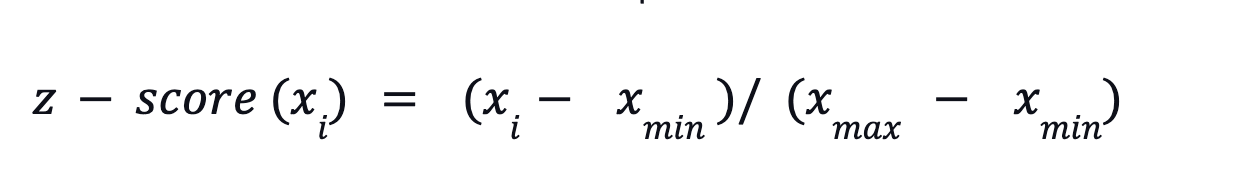The numerator is telling us how close the NFT’s average Jaccard distance is to the least rare NFT.

In the denominator, we have the difference between the maximum average distance (The rarest) and the minimum average distance. This gives us the “diversification” in an NFT collection. If the collection consists of similar elements with not a lot of rare items, the difference between the maximum and minimum value of average JDs would be quite insignificant.

This will reduce the effect of underestimating the rarity of an NFT only because of its small JD with other data points when this analogous pattern presents itself in the whole collection.

Going back to our 100 colorful NFTs example, this formula will tell us the truth about how rare our NFT is.

Stay focused on the truth

Nowadays, everyone is coming up with a new way of measuring NFT rarity. Many of these models are not understood very well by the users. My goal with this article was to give you the ultimate guide on how our rarity model actually works.

Trust is built through sharing knowledge. Now that you know the mechanism behind our rarity rankings, you can make a much more educated and precise decision when you use our Rarity metrics.

NFTGO is the digital treasury for the metaverse. We aim to give you the best experience in the transition to a new world. The truth always lies behind the data and now that you know how we approach this data to calculate rarity, you can go and explore the model and know exactly how it works.

https://nftgo.io/research/kHWaMtNY6ZOvDzr7PR99D03--VNu6-ZOjYuf6E9-QH0

Mentioned
CryptoPunks
Floor63.95
24h
-1.60%
You might be interested in
View MoreNFTGo New Feature Going Alive: The Collection Page Upgrade!

Great decisions rely on great information. Communicating key insights from data is critical to laying a successful strategy for your NFT investing journey. NFTGO crafts insightful stories of NFT data through powerful visualizations. We’re writing the next chapter of this story.

At NFTGO, we striv